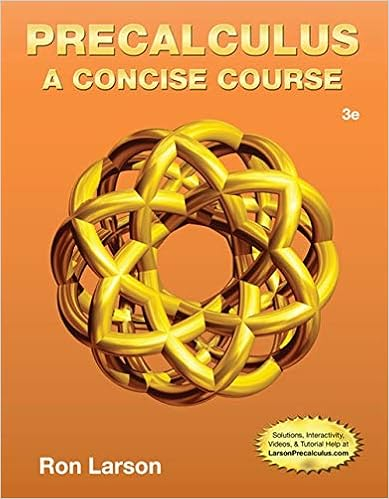# A true b false 295in a simple economy with no taxes

• 82
• 94% (34) 32 out of 34 people found this document helpful

This preview shows page 58 - 61 out of 82 pages.

##### We have textbook solutions for you!
The document you are viewing contains questions related to this textbook.The document you are viewing contains questions related to this textbook.
Chapter 2 / Exercise 94
Precalculus: A Concise Course
LarsonExpert Verified
A)TrueB)False295.In a simple economy with no taxes, government spending, exports, or imports, if disposable income increases by \$500 and \$450 is consumed, \$50 is saved.A)TrueB)False296.A \$200 million increase in investment spending will increase real GDP by exactly \$200 million.A)TrueB)False297.A \$200 million increase in investment spending will increase real GDP by more than \$200 million.A)TrueB)False298.A \$300 million decrease in investment spending will increase real GDP by more than \$300 million.A)TrueB)False299.A \$600 million decrease in investment spending will decrease real GDP by more than \$600 million.A)TrueB)False300.Autonomous spending occurs in the second round of spending increases caused by the multiplier.A)TrueB)False301.Autonomous spending is an initial change in the desired level of spending by firms, households, or government at a given level of real GDP.Page 58
##### We have textbook solutions for you!
The document you are viewing contains questions related to this textbook.The document you are viewing contains questions related to this textbook.
Chapter 2 / Exercise 94
Precalculus: A Concise Course
LarsonExpert Verified
A)TrueB)False302.If the marginal propensity to save is 0.25 in an economy with no taxes and no imports, the marginal propensity to consume is 1.25.A)TrueB)False303.If the marginal propensity to save is 0.25 in an economy with no taxes and no imports, the marginal propensity to consume is 0.75.A)TrueB)False304.In an economy with no taxes or imports, if the marginal propensity to consume increases, the marginal propensity to save will also increase.A)TrueB)False305.In an economy with no taxes or imports, if the marginal propensity to consume increases, the marginal propensity to save will decrease.A)TrueB)False306.In an economy with no taxes or imports, if disposable income increases by \$2,000 and consumption increases by \$1,400, the marginal propensity to save is 0.21.A)TrueB)False307.In an economy with no taxes or imports, if disposable income decreases by \$2,000 and consumption decreases by \$1,400, the marginal propensity to save is 0.3.A)TrueB)False308.In an economy with no taxes or imports, if disposable income decreases by \$2,000 and consumption decreases by \$1,400, the multiplier is 7.A)TrueB)FalsePage 59
309.In an economy with no taxes or imports, if disposable income decreases by \$2,000 and consumption decreases by \$1,500, the marginal propensity to consume is –0.25.A)TrueB)False310.In an economy with no taxes or imports, if disposable income decreases by \$2,000 and consumption decreases by \$1,500, the multiplier is –4.A)TrueB)False311.In an economy with no taxes and no imports, disposable income increases from \$1,000 to\$2,000. If consumption increases from \$800 to \$1500, the marginal propensity to consume is 0.7.A)TrueB)False312.In an economy with no taxes and no imports, disposable income increases from \$1,000 to\$2,000. If consumption increases from \$800 to \$1,500, the marginal propensity to save is 0.25.A)TrueB)False313.In an economy with no taxes and no imports, disposable income increases from \$1,000 to\$2,000. If consumption increases from \$800 to \$1,500, the marginal propensity to save is 0.3.
•••